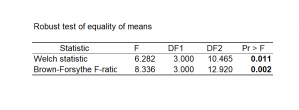# Welch und Brown-Forsythe one-way ANOVA

Welch and Brown-Forsythe ANOVA are more reliable than the classic F when variances are unequal. Both are available in Excel using the XLSTAT software.## When to use the Welch and the Brown-Forsythe ANOVA

In an analysis of variance, it may happen that the variances cannot be assumed to be equal. In this case, the F test of the ANOVA is not robust enough to be used.

XLSTAT offers two alternative tests based on the F distribution but more robust than the classical F test.

These tests are:

• Welch Test or Welch ANOVA (Welch, 1951). The Welch test adjusts the denominator of the F ratio so it has the same expectation as the numerator when the null hypothesis is true, despite the heterogeneity of within-group variance. The p-value can be interpreted in the same manner as in the analysis of variance table.
• The Brown-Forsythe test or Brown-Forsythe F-ratio (1974). This test uses a different denominator for the formula of F in the ANOVA. Instead of dividing by the mean square of the error, the mean square is adjusted using the observed variances of each group. The p-value can be interpreted in the same manner as in the analysis of variance table.

These tests are only available with a one-way Analysis of Variance.### analysieren sie ihre daten mit xlstat

kostenlose 14-tage-testversion

Enthalten ind

Verwandte Funktionen